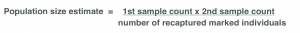Biology 31/10/2018

# Maths skills in GCSE Biology

By KATIE ROSS

In the GCSE Biology exam, approximately 10% of the questions will require you to apply maths skills. This blog provides an overview of the main maths skills on which you will be assessed.

This blog provides an overview. For much more detail, with explanations and opportunities for practice, have a look at the My GCSE Science video tutorials covering maths skills in Biology.

Go to the My GCSE Science search area and enter the term “maths skills”. This will return all the relevant videos. Below the tutorials you can find exam-style questions where you can apply the knowledge you’ve learnt from the tutorials. You can also find mark schemes, so that you can assess your progress!

## MagnificationYou need to be able to rearrange this equation. For example, in the exam you may be given the value of magnification and the actual size and then be asked to calculate the image size. In this case, you will need to measure the drawing (across its widest length) on the exam paper itself to get a value for the image size. So please don’t forget to bring a ruler to the exam!

Cells are often measured in micrometres (mm). Always measure the image size in mm with your ruler, then multiply this by 1000 to convert the measurement to mm.

## Estimates

In the exam, you may need to estimate the size of a cell. There are two ways of doing this.

### Estimate the size of a cell – Method 1

This method works best under lower magnifications. Measure the field of view of your microscope under a certain magnification. Place a ruler on the microscope stage and count the number of mm seen on the ruler to find the diameter of the field of view. Multiply the number of mm by 1000 to convert to mm. Now count how many cells you can see across your field of view. Finally, divide the length of the diameter by the number of cells seen. This provides an estimate of the size of each cell.

### Estimate the size of a cell – Method 2

A more accurate estimate of cell size can be found by using an eyepiece graticule. A graticule is a ruler (with no numbers) in the eyepiece of the lens. In the exam, you may be provided with the value (in mm) of one eyepiece graticule unit. Or you may be asked to calculate the value of one eyepiece graticule unit, based on how many of these units fit within a measurement on a stage micrometre (a ruler with numbers).  Once you know the value (in mm) of each eyepiece graticule unit, count how many units fit along the widest part of a cell. Multiply the number of units by the value (in mm) of one unit.

### Estimate the population of a stationary species

In the Biology GCSE exam, you may be asked to estimate the population of a species in a habitat.

For an estimate of the number of a plant species in a field, follow the steps below:

1. Count the number of individuals of a species in each of 10 quadrats.
2. Calculate the total number of individuals in Step 1 and then divide by 10 to work out the average number of the species in each quadrat.
3. Measure the area of the field (length x width) and then the area of a quadrat.
4. Calculate how many quadrats fit on the field (area of field / area of quadrat).
5. Multiply the number of quadrats in Step 4 by the average number of the species you calculated in Step 2.

For more explanations of the terms used above, see the video tutorial Measuring the Distribution of Organisms (which covers AQA Required Practical 9).

### Estimate the population of a moving species (OCR only)

If you are following the OCR specification, you may be asked to estimate the population of a moving species. In this case, the “capture-mark-release and recapture” method is used and the following equation applied. For more on this see the OCR video on Sampling Techniques.## Percentage change

The percentage change calculation is important in the context of the osmosis practical that is a requirement of all exam boards. Apply the following formula:## Efficiency

An efficiency calculation is required in the context of biomass transfer through food chains. Use the following equation:## Area of a circle

This equation (which you know from Maths) will be necessary when analysing inhibition zones caused by antibiotics or antiseptics on bacterial growth. Pi (π) can be estimated at 3.14 and r is the radius of a circle (half the diameter).

Area of a circle = π r 2

## Calculating the rate of reaction

The rate of biological reactions can be calculated in a number of ways.

To calculate the rate of transpiration, you could measure the distance a bubble moves in a potometer over time.

Alternatively, you could measure the speed of product formation. For example, the rate of photosynthesis can be calculated by measuring the volume of oxygen produced over time or the number of oxygen bubbles produced over time.

A trickier one is calculating the rate of lipase-controlled reactions. Here, the product fatty acid leads to a drop in pH and therefore the change in pH over time can be used to work out the rate of reaction.

You could measure the speed of reactant use, for example, by measuring a change in mass over time, including the drop in mass of compost material in decay reactions.

In all cases, the units for the rate will depend on the units used for time and the other variable.

That’s it! Remember your ruler, keep a cool head and best of luck!

Katie Ross